• 如果y是实数矩阵，则相当于对y的每一列进行二维绘图。 如果y是复数组成的向量，则相当于plot(real(y),imag(y))。 2、plot(x,y) 当x和y都是矢量，如果其长度相等，则以x为横坐标，y为纵坐标。 当x为矢量，y是有一...
基本绘图函数

1、plot(y)

如果y是向量，则采用向量的索引值作为横坐标值，以向量元素的值作为纵坐标值。

如果y是实数矩阵，则相当于对y的每一列进行二维绘图。

如果y是复数组成的向量，则相当于plot(real(y),imag(y))。

2、plot(x,y)

当x和y都是矢量，如果其长度相等，则以x为横坐标，y为纵坐标。

当x为矢量，y是有一维与x同维的矩阵时，则以x为共同的横坐标绘制多条不同颜色的曲线，曲线的条数由y的另一维决定。

当x，y是同维的矩阵时，则以x和y对应的列元素为横纵坐标分别绘制曲线，曲线条数等于矩阵的列数。

clear all;
x=1:7;
y=magic(7);
plot(x,y);

clear all;
x=1:7;
y=magic(7);
plot(x,y)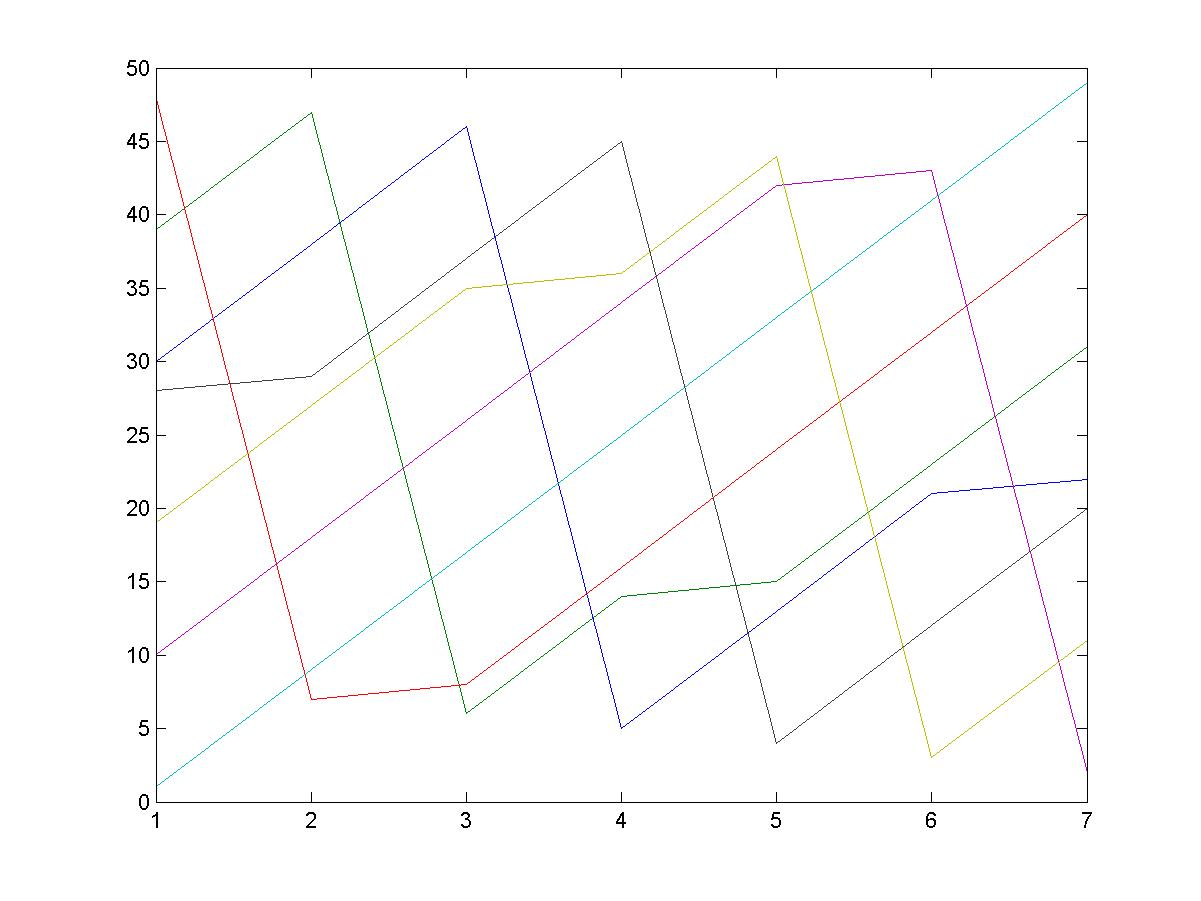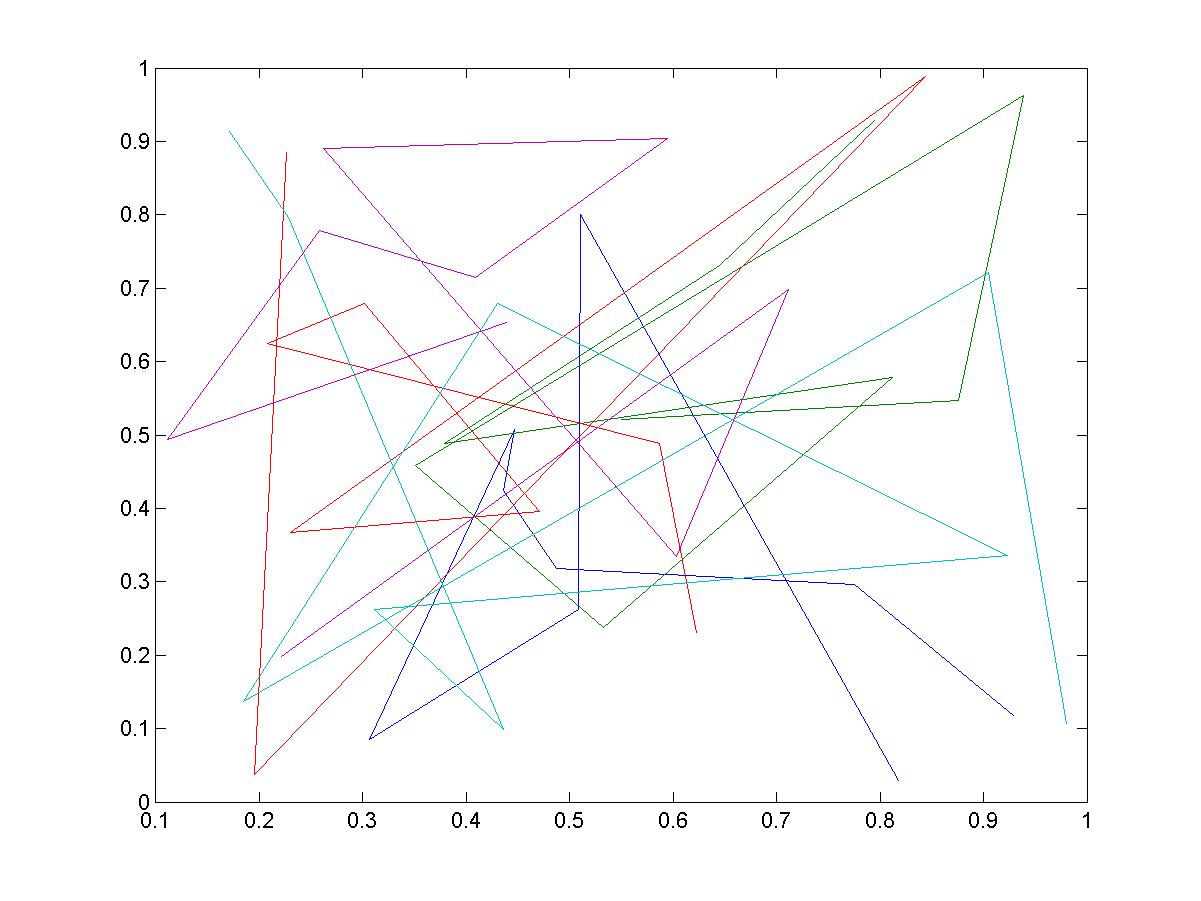3、plot(x,y,s)

s是对二维绘图的线型和颜色等进行设置。

二维绘图常用设置
线型
说明
标记符
说明
颜色
说明
-
实线（默认）
+
加号符
r
红色
--
虚线
o
空心圆
g
绿色
：
点线
*
星号
b
蓝色
-.
点划线
.
实心圆
c
青绿色

x
叉号符
m
洋红色

s
正方形
y
黄色

d
菱形
k
黑色

^
上三角形
w
白色

v

下三角形

>
右三角形

<
左三角形

p
正五边形

h
六边形

clear all;
x=0.01:0.3:2*pi;
y=cos(x+0.5)+2;
subplot(2,2,1);
plot(x,y,'k');
subplot(2,2,2);
plot(x,y,'k.');
subplot(2,2,3);
plot(x,y,'k-^');%表示用黑色的实线（默认）连接各点，各点采用上三角标记。
subplot(2,2,4);
plot(x,y,'k^');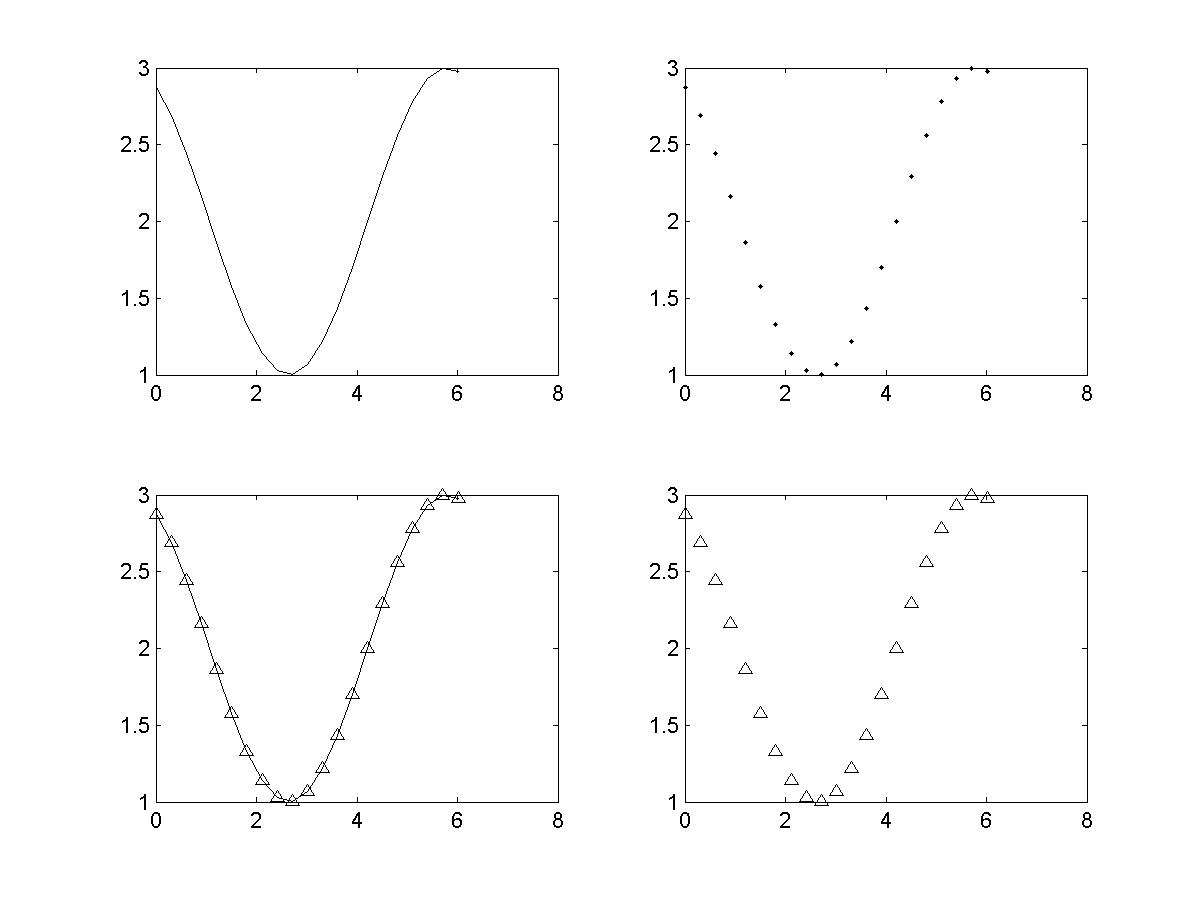函数plot()绘图的PropertyName
PropertyName
说明
取值
LineWidth
线宽
数字，单位是points
MarkerEdgeColor
标记点边框的颜色
颜色字符
MarkerFaceColor
标记点内部填充颜色
颜色字符
MarkerSize
标记点大小
数字，单位是points
%如左下图
clear all;
x=-pi:pi/10:pi;
y=tan(sin(x))-sin(tan(x));
plot(x,y,'--rs','LineWidth',2,'MarkerEdgeColor','k','MarkerFaceColor','g','MarkerSize',10);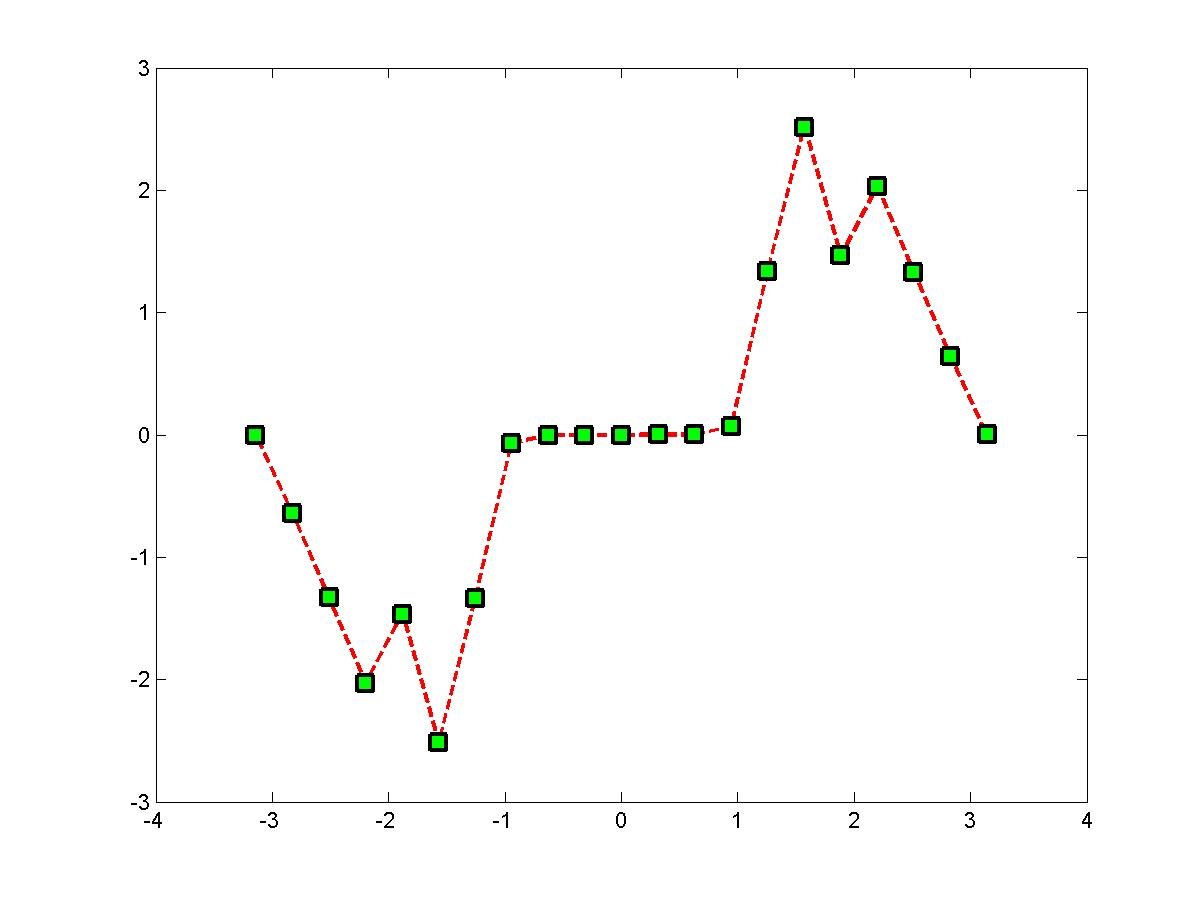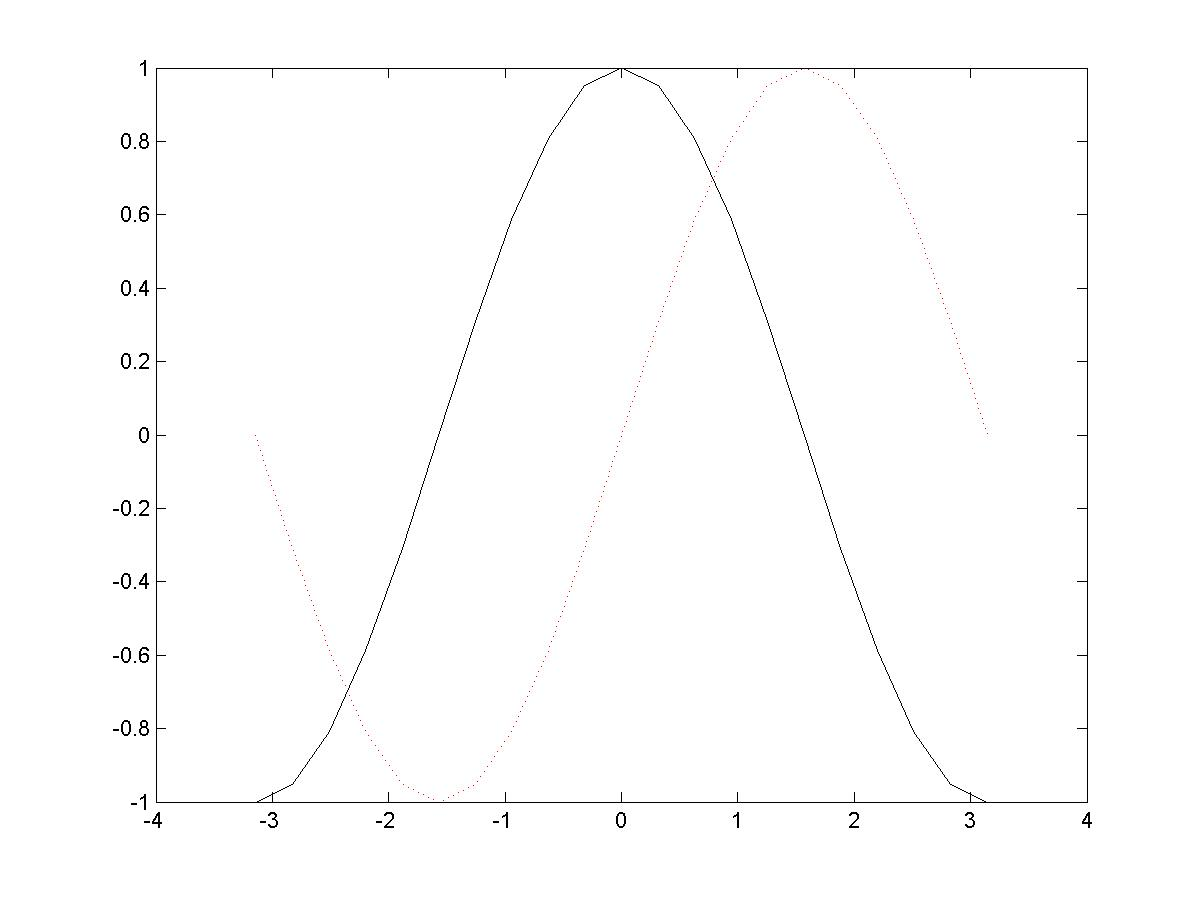4、plot(x1,y1,s1,x2,y2,s2,...)

%如右上图
clear all;
x=-pi:pi/10:pi;
y=sin(x);
z=cos(x);
figure;
plot(x,y,'r:',x,z,'k-');

子图绘制

subplot进行子图绘制。

subplot(m,n,p)：该函数将当前窗口分成m x n个绘图区。子绘图区的编号按行优先从左到右编号。
clear all;
x=-pi:pi/10:pi;
figure;
subplot(221);
plot(x,sin(x),'r--');
subplot(223);
plot(x,cos(x),'b:*');
subplot(2,2,[2,4]);
plot(x,sin(x)+cos(x),'b-.^');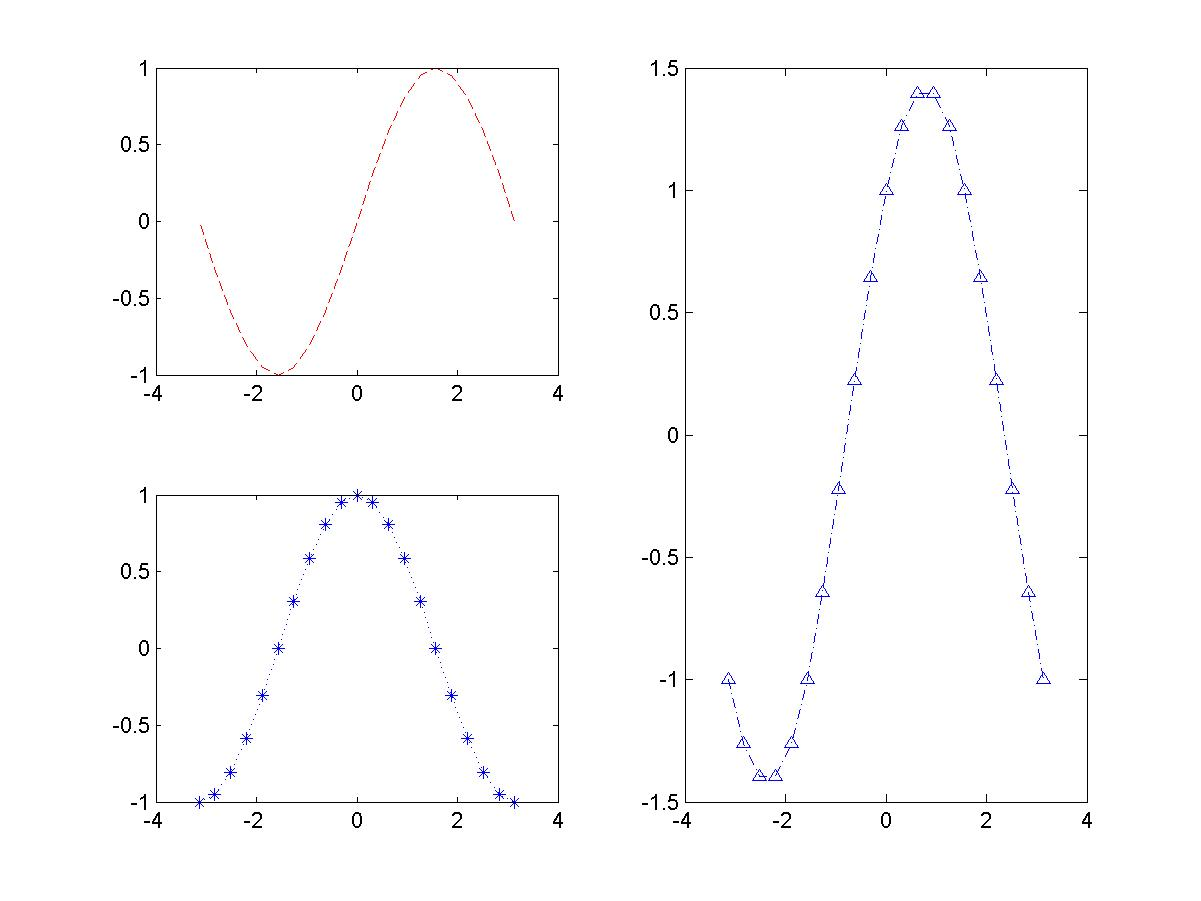叠加图绘制

hold函数启动或关闭图形保持功能。

hold on：启动图形保持功能，允许在同一坐标轴上绘制多个图
hold off：关闭图形保持功能。
hold：在hold on 和 hold off之间切换。
设置坐标轴

axis([xmin xmax ymin ymax])
axis([xmin xmax ymin ymax zmin zmax])
axis off ：取消坐标轴的一切设置
axis on ：恢复坐标轴的一切设置
网格线和边框

通过函数gird()给坐标轴加网格线

grid on ：给当前坐标轴添加网格线
grid off ：取消当前坐标轴的网格线
grid ：在grid on 和 grid off 之间切换
clear all;
x=-pi:pi/10:pi;
figure;
plot(x,sin(x)+cos(x),'b-.^');
grid on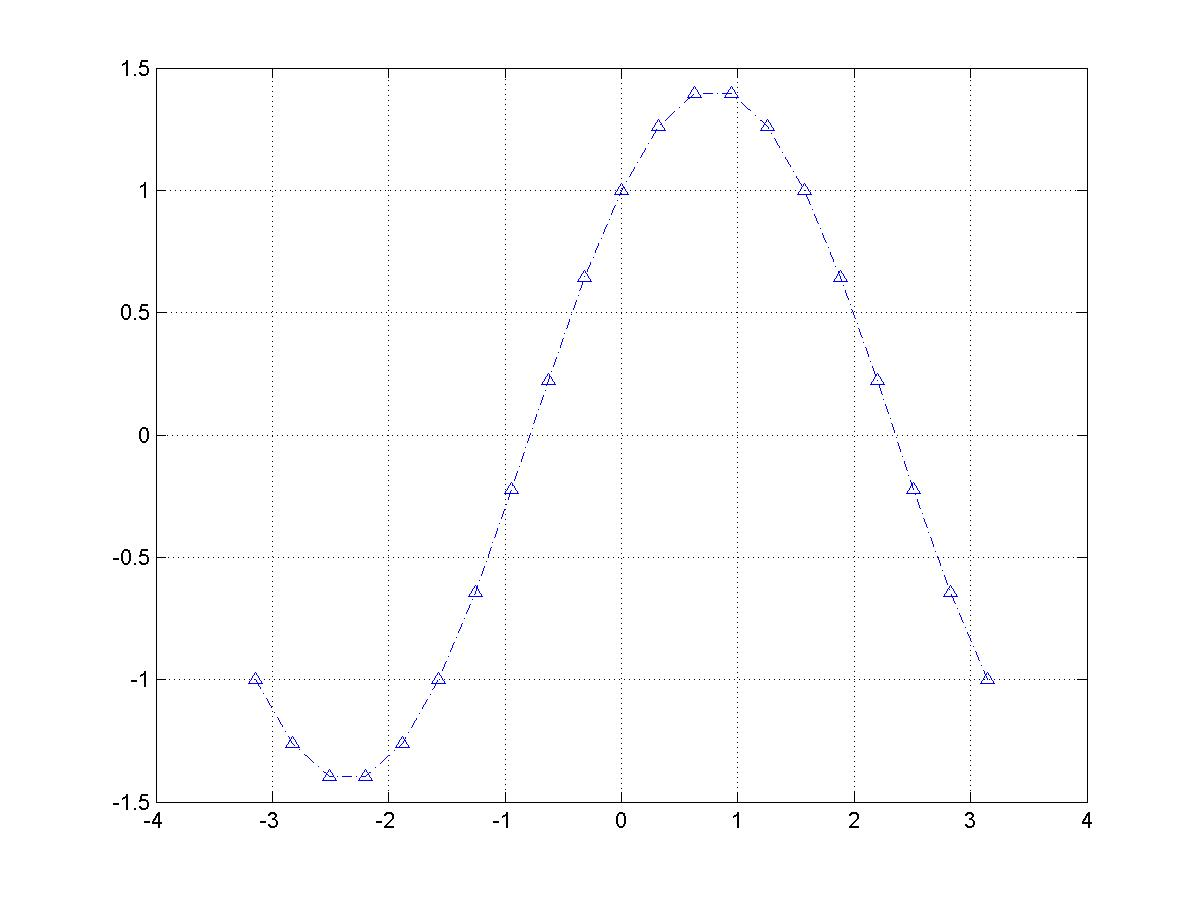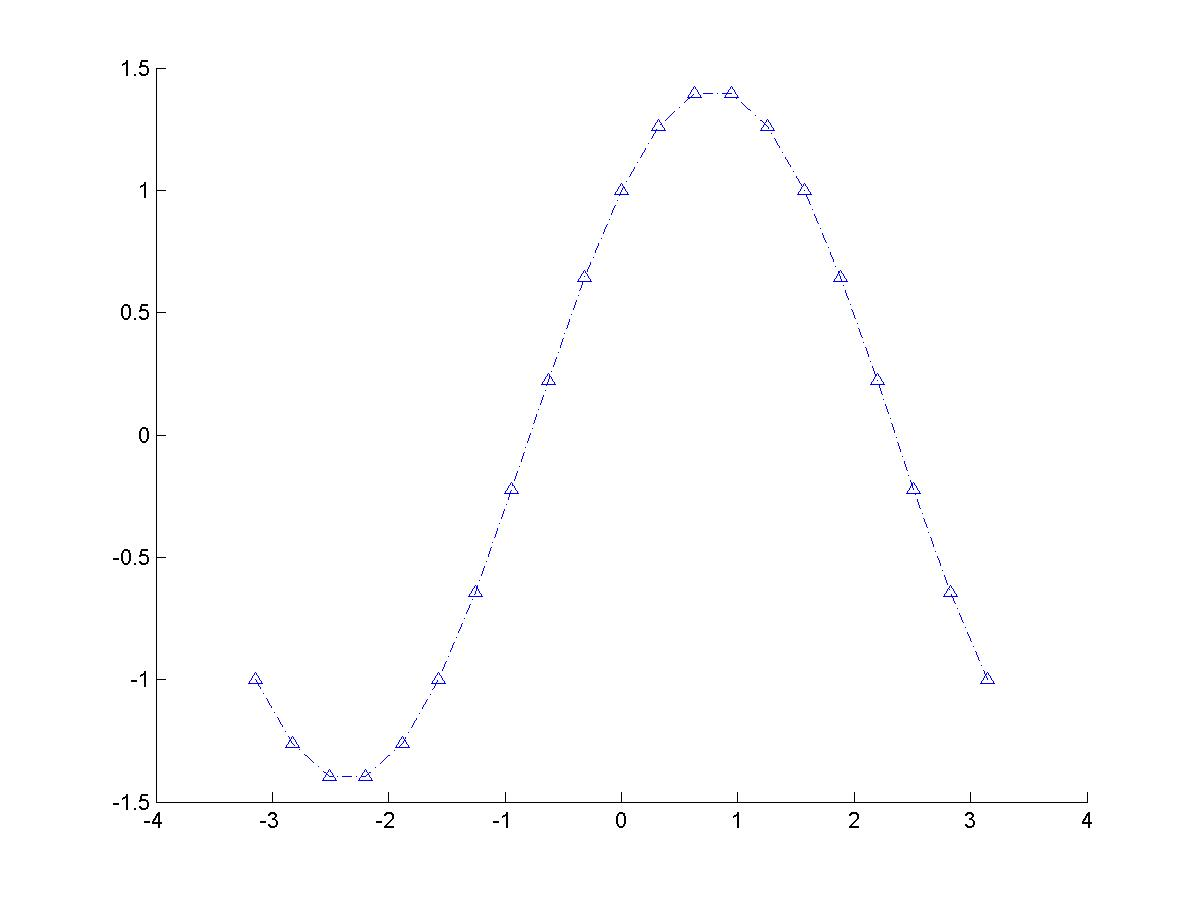box()函数给坐标轴加边框

box on ：该函数给当前坐标轴添加框线。
box off ：该函数取消当前坐标轴的边框线。
box ：不带参数时，在box on 和box off 之间切换。
%见右上图
clear all;
x=-pi:pi/10:pi;
figure;
plot(x,sin(x)+cos(x),'b-.^');
box off

数据光标

datacursormode()显示鼠标所选的图形上某点的坐标值。

datacursormode on ：该函数打开图形的数据光标功能。
datacursormode off ： 该函数关闭图形的数据光标功能。
datacursormode：在datacursormode on 和 datacursormode off 之间转换。
%见左下图
clear all;
x=0.1:0.1:2*pi;
y=sin(x);
figure;
plot(x,y,'--r');
datacursormode on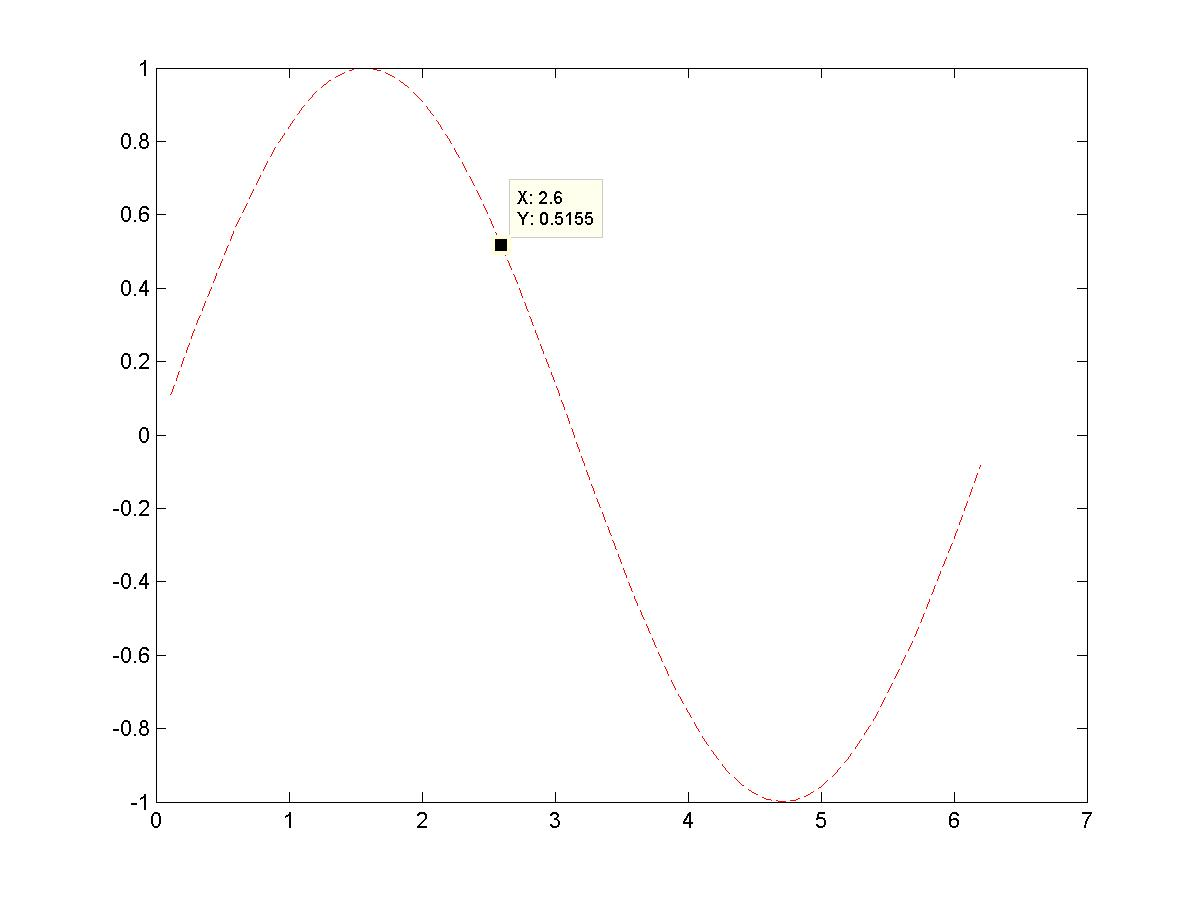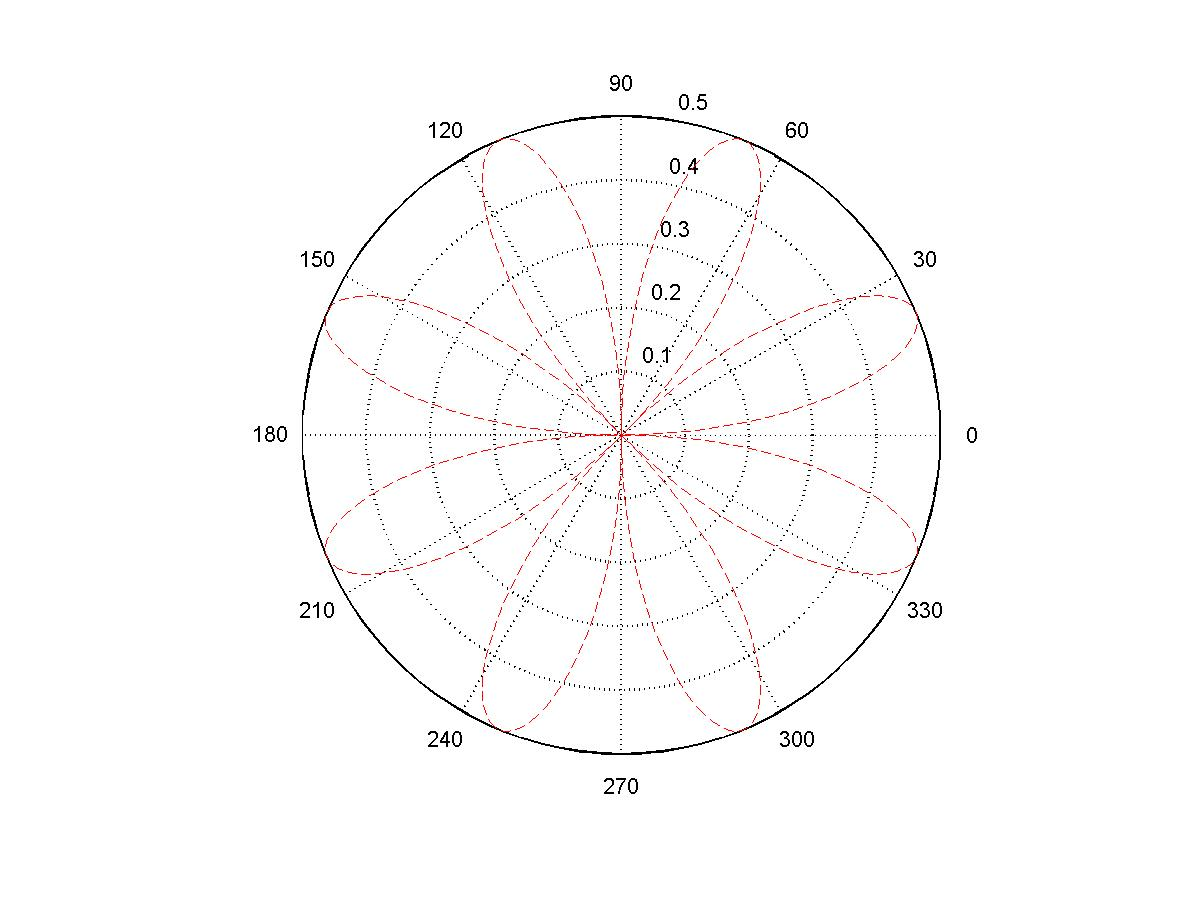极坐标绘图

polar(theta,rho)：弧度为theta，半径为rho
polar(theta,rho,s)：参数s对曲线的线型、标记和颜色等信息进行设置。
%见右上图
clear all;
x=0:0.02:2*pi;
y=sin(2*x).*cos(2*x);
figure;
polar(x,y,'--r');

[x,y]=pol2cart(theta,rho)：将极坐标转换为直角坐标
[x,y,z]=pol2cart(theta,rho,Z)
[theta,rho]：cart2pol(x,y)：将直角坐标转换为极坐标
[theta,rho,Z]：cart2pol(x,y,z)
clear all;
theta=0:pi/40:4*pi;
rho=sin(theta);
figure;
subplot(211);
polar(theta,rho);
[x,y]=pol2cart(theta,rho);
subplot(212);
plot(x,y);
axis equal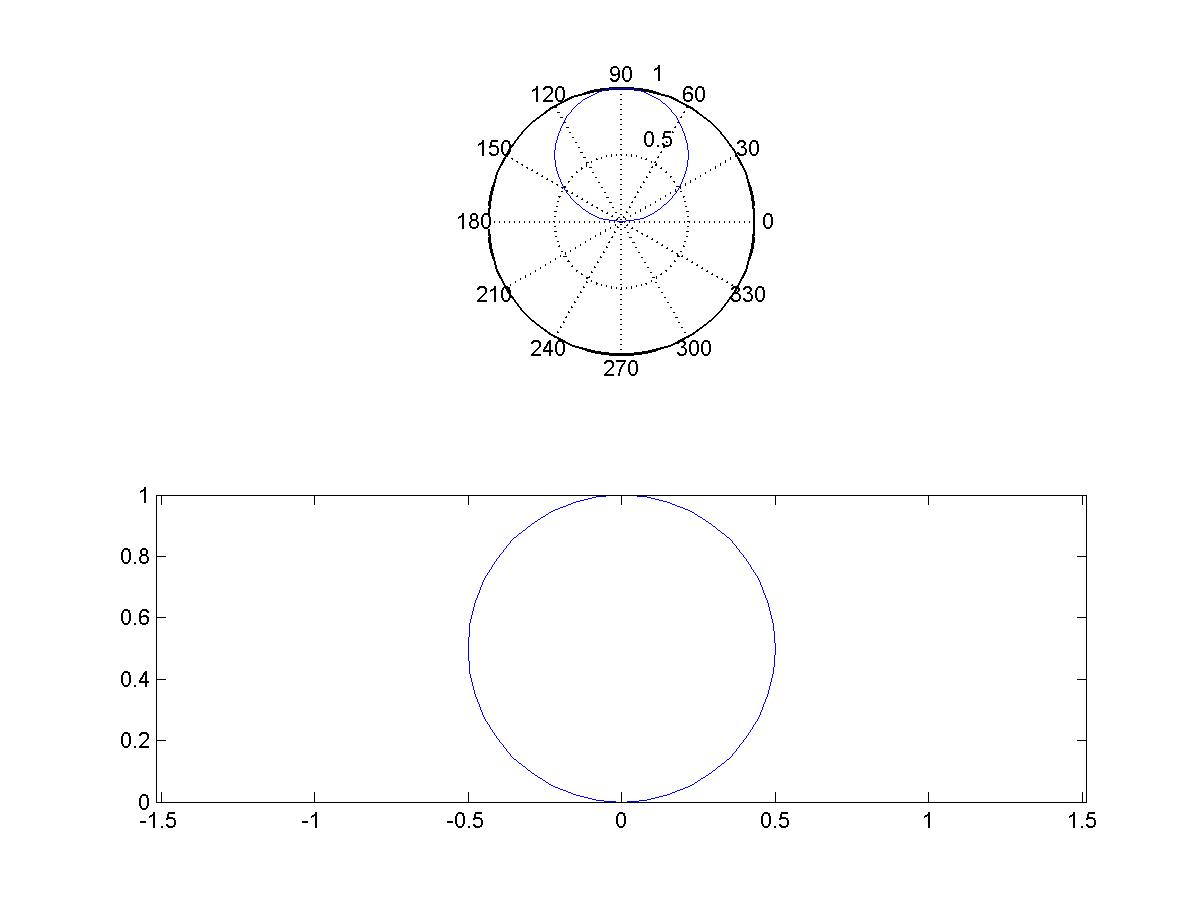对数和半对数坐标系绘图

semilogx()：x轴采用对数坐标，y轴采用等比例刻度坐标。

semilogy()：y轴采用对数坐标，x轴采用等比例刻度坐标。

loglog()：x，y 轴采用对数坐标。

双纵轴绘图

plotyy(x1,y1,x2,y2)：(x1,y1)采用左侧y轴，(x2,y2)采用右侧y轴
plotyy(x1,y1,x2,y2,'function')：function可以是"plot","semilogx","semilogy","loglog"
plotyy(x1,y1,x2,y2,'function1','function2')：function1绘制(x1,y1)，function2绘制(x2,y2)
clear all;
x=0.1:0.1:2*pi;
y=sin(x);
z=10.^x;
figure;
plotyy(x,y,x,z,'plot','semilogy');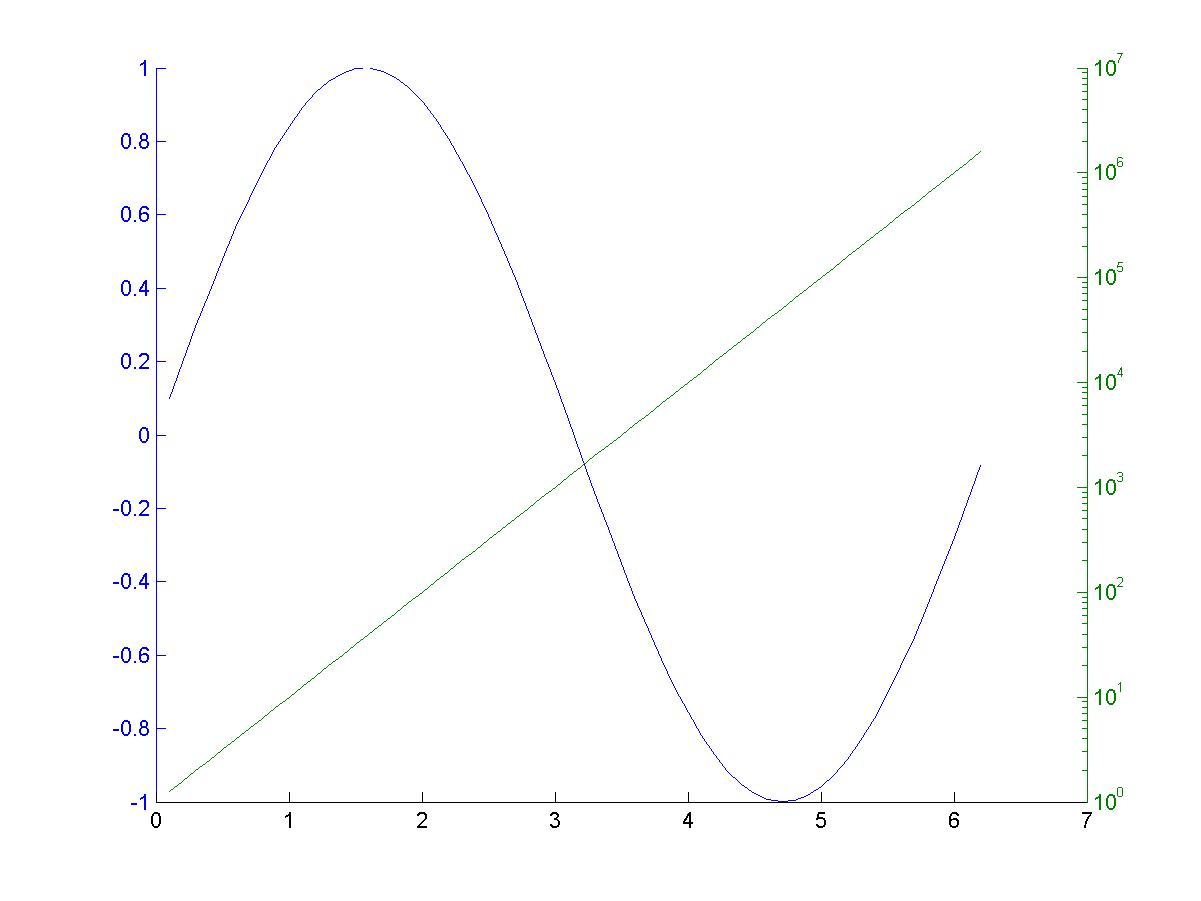展开全文• 矩阵绘图 %% 3.绘制三条曲线 %% 4.绘制双纵坐标图 %% 5.极坐标绘图 %% 6.极坐标和直角坐标的相互转化 %% 7.对数坐标系绘图 %% 8.图形窗分割 subplot() %% 9.坐标轴设置 %% 10.图形标识 %% 11.ezplot()简易绘图指令...
%% 1.基本绘图指令plot()
%% 2.矩阵绘图
%% 3.绘制三条曲线
%% 4.绘制双纵坐标图
%% 5.极坐标绘图
%% 6.极坐标和直角坐标的相互转化
%% 7.对数坐标系绘图
%% 8.图形窗分割 subplot()
%% 9.坐标轴设置
%% 10.图形标识
%% 11.ezplot()简易绘图指令(用于隐含数绘图)
%% 12.ezploar()极坐标图形简易绘制
%% 13.直方图绘制
%% 14.扇形图绘制
%% 15.离散杆状图和离散阶梯图
%%
clf;%用于清除图形，一般在画图前使用
% 1.基本绘图指令plot()
x1=linspace(0,4,10);
y1=sin(x1)+sin(8*x1);
x2=linspace(0,4,100);
y2=sin(x2)+sin(8*x2);
subplot(1,2,1);%在同一个窗口中创建一行两列区域在第 1 个区域显示图形
plot(x1,y1);%绘图指令，x1为横坐标，y1为纵坐标
title('picture1');%图形名称
subplot(1,2,2);%第 2 个区域显示图形
plot(x2,y2,x2,y2,'r*');%前两个x2,y2用来显示曲线连接，后两个x2，y2用来显示*，如果没有前两个则直接为散点 *
title('picture2');
% 2.矩阵绘图
x=linspace(0,1,30)';%列向量
k=linspace(0.2,0.8,7);%行向量
y=exp(0.5*x)*k;%30*7的矩阵
plot(y,x);%显示7根曲线
% 3.绘制三条曲线
x=linspace(0,6,20);
y=x.^2.*sin(x);%每个元素独立进行标量运算，需要带上点（否则会因为维数不同而报错）
figure(1);%新建 1 图形窗口 1
plot(x,y);
figure(2);%新建图像窗口（避免被刷新）
y1=y+2;
y2=y-2;
plot(x,y,x,y1,'k-.*',x,y2,':x');%在一幅图中显示 3 个图像，依次类推 n
% 4.绘制双纵坐标图
x=linspace(0,8,100);
y1=2*exp(-0.5*x).*sin(2*pi*x);
y2=4.5*exp(-0.1*x).*sin(x);
plotyy(x,y1,x,y2);%绘制双纵坐标图
% 5.极坐标绘图
th=linspace(0,2*pi,100);
r=3*(1+cos(th));
polar(th,r,'g-*');%极坐标指令，依次为θ、ρ、控制符
% 6.极坐标和直角坐标的相互转化
[th,r]=cart2pol(1,3);%直角坐标转换为极坐标
[x,y]=pol2cart(pi/3,2);%极坐标转化为直角坐标
% 7.对数坐标系绘图
x=linspace(0,10,100);
y=x.^3+x.^2-2*x+6;
figure(1);
semilogy(x,y);%半对数坐标（y）
grid on;      %显示网格
figure(2);
semilogx(x,y);%半对数坐标（x）
grid on;
figure(3);
loglog(x,y);%全对数坐标
grid on;
% 8.图形窗分割 subplot()
subplot(2,3,1:2);%分区后将 1、2 区域合并
t=linspace(0,10,200);
y1=sin(2*pi*t);
plot(t,y1);
subplot(233);%与subplot(2，3，3)等价
y2=sin(t);
plot(t,y2);
subplot('position',[0.2 0.05 0.65 0.45]);%左下角（0.2,0.05）、右上角（0.65,0.45）
y3=y1.*y2;
plot(t,y3,'g-',t,y2,':r',t,-y2,':r');
% 9.坐标轴设置
x=linspace(0,2*pi,30);
y=sin(x).*cos(2*x);
plot(x,y,'g');
hold on;%在当前图形上继续画图
plot(x,y+0.5);
hold off;
axis([0,2*pi,-1.5,1.5]);%设置坐标系统的最大值和最小值
set(gca,'xtick',linspace(0,5,6),'ytick',[-1.5,0,0.2,1]);%设定坐标轴的刻度标识
% 10.图形标识
t=0:pi/100:2*pi;
y1=2*sin(2*t);
y2=3*sin(3*t);
plot(t,y1,t,y2,'g-.');
axis([0,pi,-4,4]);
title('正弦曲线0\rightarrow\pi');%图形标题
xlabel('时间');%图形横坐标
ylabel('函数值');%图形纵坐标
text(pi/4,2*sin(pi/2),'pi/4的函数值');%文本
gtext('3sin(3t)的极小值');%鼠标确定文本位置
legend('2sin(2t)','3sin(3t)','NorthEast')%输出图形图例，最后一个参数表示方位
% 11.ezplot()简易绘图指令(用于隐含数绘图)
subplot(2,2,1);
ezplot('x^2-y+1');%f(x)=x^2-y+1,直角坐标形式，此处缺省为默认区间(x,y)∈[-2pi,2pi]
subplot(222);
ezplot('x^2-cos(y)+sin(x)',[-2,1,-15,15]);%此处没缺省,区间为x∈(-2,1)，y∈(-15,15)
subplot(223);
ezplot('exp(t)*sin(t)','exp(t)*cos(t)');%x(t)=exp(t)*sin(t),y(t)=exp(t)*cos(t) 参数方程形式，此处缺省为默认区间t∈(0,2pi)
subplot(224);
ezplot('sin(t)','cos(2*t)',[-8,6]);%此处没缺省,区间为t∈(-8,6)
% 12.ezploar()极坐标图形简易绘制
ezpolar('abs(2*cos(2*(t-pi/8)))');%第一个参数为ρ，第二个参数缺省时为t∈(0,2pi)
ezpolar('abs(2*cos(2*(t-pi/8)))',[0,4/3*pi]);%第二个参数没缺省，t∈(0,4/3pi)
% 13.直方图绘制
y=round(rand(5,3)*10);
subplot(1,3,1);
bar(y,'group');% bar 垂直分组
title('垂直分组式');
legend('first','second','third');
axis([0,6,0,12]);
xlabel('x');
ylabel('y');
subplot(132);
bar(y,'stack');% bar 垂直积累
title('垂直积累式');
axis([0,6,0,25]);
xlabel('x');
ylabel('y');
subplot(133);
barh(y,0.5);% barh 水平显示
title('水平分组式，宽度0.5');
xlabel('x');
ylabel('y');
% 14.扇形图绘制
data=[10 12 19 30 42];% 数据
explode=[0 1 0 0 100];% 对应数据在扇形图中所占的部分是与总体否分开，分开的大小
pie(data,explode);
% 15.离散杆状图和离散阶梯图
t=linspace(0,2*pi,20);
y=cos(t).*exp(-0.2*t);
stem(t,y,'filled');%离散杆状图 最后一个参数缺省时表示空心，filled表示实心
title('离散信号的杆图和阶梯图');
xlabel('t');
ylabel('cos(t)*e^{-0.2t}');
hold on;
stairs(t,y,'g:');%离散阶梯图
hold off;
展开全文• 需要求多个峰值的二维矩阵如下： figure(); % 总功率谱绘图 surf(MatrixA); 绘图结果： 然后求取峰值位置（需要安装图像处理工具箱）： PeaksMap = imregionalmax(MatrixA); 其得到的结果如下，可以看到是峰值的...
• 基本绘图命令（一）基本绘图函数介绍1、plot(y) 向量或者实数矩阵。对于实数向量，以向量索引为横坐标，向量值为纵坐标；复数向量，实部为横坐标，虚部为纵坐标；对于实数矩阵，该函数对每一列绘图，有多少列，就有...
基本绘图命令（一）基本绘图函数介绍1、plot(y)      向量或者实数矩阵。对于实数向量，以向量索引为横坐标，向量值为纵坐标；复数向量，实部为横坐标，虚部为纵坐标；对于实数矩阵，该函数对每一列绘图，有多少列，就有多少曲线。2、plot(x,y)   x,y都为向量，x为横坐标，y为纵坐标；如果x是向量，y是矩阵，    要求y至少有一个维度与x相同，以x为横坐标，y为纵坐标；x,y都为矩阵，则以x,y每一列对应的元素为横纵坐标。3、plot(x,y,s)   s是对绘图的一些参数设置，包括颜色、线型和数据点的标记。还可以对线宽等进行设置。4、plot(x1,y1,s1,x2,y2,s2,……)可以同时绘制多条曲线。常用的颜色和线性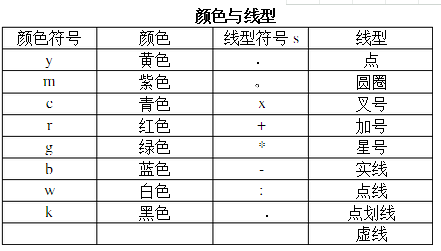（二）基本绘图设置1、叠加图hold on   启动图形保持功能。hold off   关闭图形保持功能。hold      在on和off之间切换。hold all   实现on的功能，并使新的绘图函数按顺序循环使用当前坐标系中的colorOeder          和LineStyleOrder。2、设置坐标轴axis([x1 x2 y1 y2])                      二维图形的横纵坐标。axis off                                       取消坐标轴显示。axis on                                       恢复坐标轴的一切设置。axis equal                                  横纵轴采取等长刻度axis square                                产生正方形坐标系axis auto                                   使得坐标轴的范围能够容纳所有图形。axis normal                               解除对坐标轴的一切设置3、网格线和边框（1）网格线       grid on                      设置网格线                   grid off                      取消网格线设置       grid                           在on和off之间切换       grid minor                 设置小网格（2）边框        box on                      设置边框        box off                      取消边框        box                            在on和off之间切换4、设置标题图形标题title（“”，Name，Value）横纵坐标轴标题xlabel(“”, Name，Value)ylabel(“”, Name，Value) 常用参数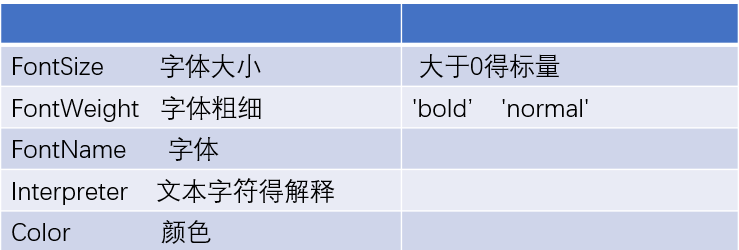标题常用颜色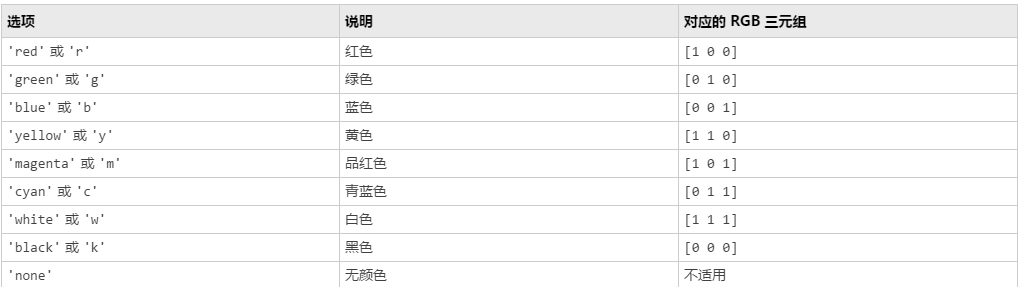5、添加图例legend(“xx1”,”xx2”,”xx3”……)          添加图例legend(‘off’)                       清除图例legend(‘toggle’)                    显示和清除间切换legend(‘hide’)                      隐藏legend(‘show’)                     显示legend(‘boxoff’)                    隐藏图例边框legdend(‘boxon’)                   显示图例边框高级绘图命令子图的绘制subplot(m,n,p) 函数m,n代表将图分为几个子图，p是子图的标号，表示在那个子图进行操作。坐标轴的缩放zoom(factor)                 以f作为缩放因子进行坐标轴的缩放 zoom on                        使能缩放zoom off                        取消缩放zoom                             on和off之间切换zoom out                      恢复到最初的坐标轴设置。zoom reset                    对当前坐标轴进行复位zoom xon                      x轴缩放zoom yon                      y轴缩放图形拖拽pan on                          使能图形拖拽pan xon                         使能图形x轴拖拽pan yon                         使能图形y轴拖拽pan off                          取消图形拖拽pan                             在on和off之间切换4、数据光标datacursormode on                    使能数据光标 datacursormode off                    取消数据光标datacursormode                         在on和off之间切换极坐标polar(theta,rho,s):pol2cart()    极坐标中的坐标值转换成直角坐标。cart2pol()   直角坐标中的坐标值转换成极坐标.对数和半对数坐标semilogx()    x轴对数,y轴等比例semilogy()loglog()双纵坐标plotyy(x,y1,x,y2)            公用一个横坐标，左右两个纵坐标。plotyy(x,y1,x,y2，‘plot’,’semilogy’)     可以设置其中一个或者两个为对数坐标获取和显示数据点[x y]=ginput(n)    鼠标选择某个点，坐标值返回到向量中。 [x y]=ginput        多个点，Enter键结束[x y button]=ginput(…….)             记录鼠标点击时的信息。三、特殊图形的绘制1、柱状图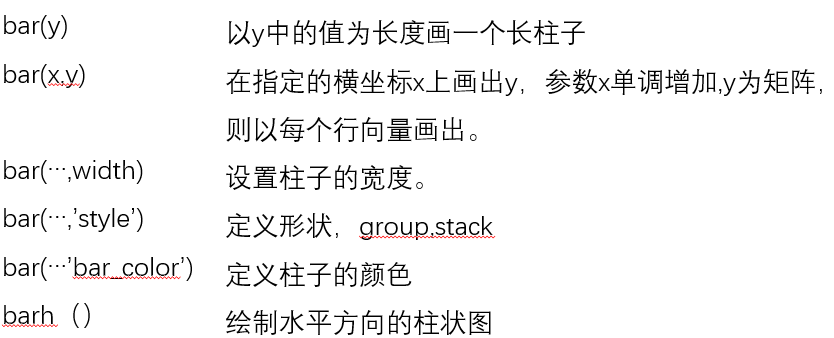饼状图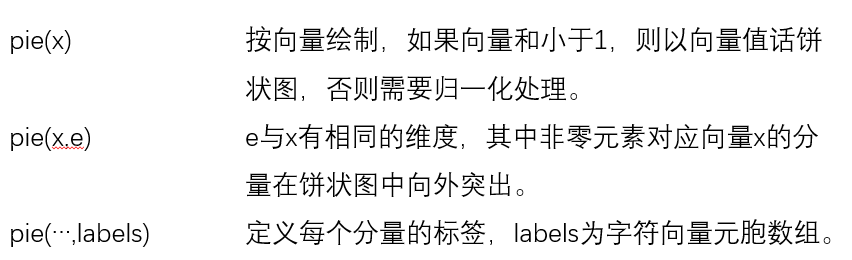直方图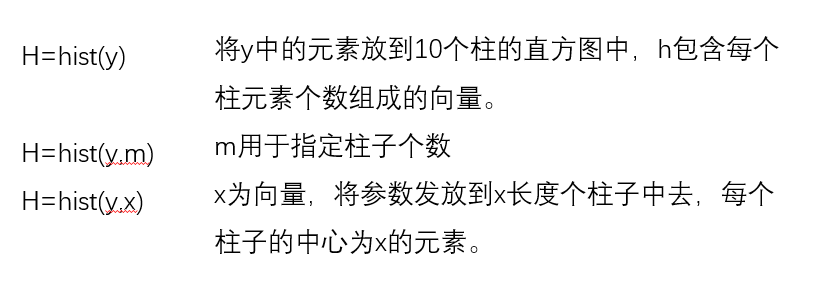散点图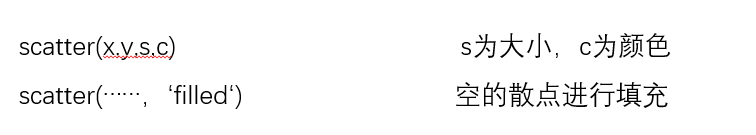5、其他不常用图形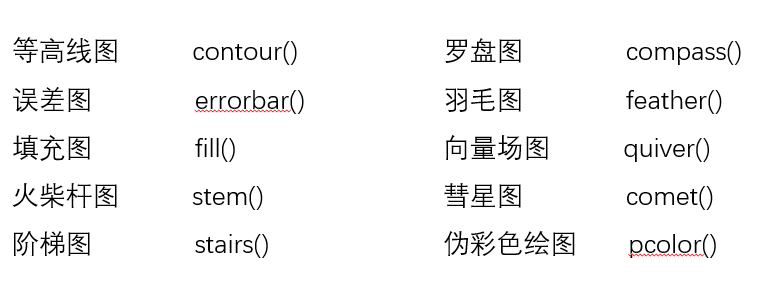展开全文• 下面将介绍MATLAB基本xy平面及xyz空间的各项绘图命令，包含一维曲线及二维曲面的绘制、列印及存档。 plot 是绘制一维曲线的基本函数，但在使用此函数之前，我们需先定义曲线上每一点的x及y座标。 下例可画出一条...
MATLAB不但擅长於矩阵相关的数值运算，也适合用在各种科学目视表示（Scientific visualization）。下面将介绍MATLAB基本xy平面及xyz空间的各项绘图命令，包含一维曲线及二维曲面的绘制、列印及存档。

plot

是绘制一维曲线的基本函数，但在使用此函数之前，我们需先定义曲线上每一点的x及y座标。

下例可画出一条正弦曲线：

close all; x=linspace(0, 2*pi, 100); % 100个点的x座标
y=sin(x); % 对应的y座标
plot(x,y);

MATLAB基本绘图函数

plot: x轴和y轴均为线性刻度（Linear scale）
loglog: x轴和y轴均为对数刻度（Logarithmic scale）
semilogx: x轴为对数刻度，y轴为线性刻度
semilogy: x轴为线性刻度，y轴为对数刻度

画出多条曲线：

只需将座标对依次放入plot函数即可：
plot(x, sin(x), x, cos(x));
若要改变颜色，在座标对后面加上相关字串即可：
plot(x, sin(x), 'c', x, cos(x), 'g');
若要同时改变颜色及图线型态（Line style），也是在座标对后面加上相关字串即可：
plot(x, sin(x), 'co', x, cos(x), 'g*');

plot绘图函数的叁数

字元 颜色 字元 图线型态
y 黄色 . 点
k 黑色 o 圆
w 白色 x x
b 蓝色 + +
g 绿色 * *
r 红色 - 实线
c 亮青色 : 点线
m 锰紫色 -. 点虚线
-- 虚线



图形完成后，我们可用axis([xmin,xmax,ymin,ymax])函数来调整图轴的范
围：
axis([0, 6, -1.2, 1.2]);
此外，MATLAB也可对图形加上各种注解与处理：
xlabel('Input Value'); % x轴注解
ylabel('Function Value'); % y轴注解
title('Two Trigonometric Functions'); % 图形标题
legend('y = sin(x)','y = cos(x)'); % 图形注解
grid on; % 显示格线

我们可用subplot来同时画出数个小图形於同一个视窗之中：

subplot(2,2,1); plot(x, sin(x));
subplot(2,2,2); plot(x, cos(x));
subplot(2,2,3); plot(x, sinh(x));
subplot(2,2,4); plot(x, cosh(x));


其他各种二维绘图函数

bar 长条图
errorbar 图形加上误差范围
fplot 较精确的函数图形
polar 极座标图
hist 累计图
rose 极座标累计图
stairs 阶梯图
stem 针状图
fill 实心图
feather 羽毛图
compass 罗盘图
quiver 向量场图

当资料点数量不多时，长条图是很适合的表示方式：

close all; % 关闭所有的图形视窗
x=1:10;
y=rand(size(x));
bar(x,y);

如果已知资料的误差量，就可用errorbar来表示：
下例以单位标准差来做资料的误差量：

x = linspace(0,2*pi,30);
y = sin(x);
e = std(y)*ones(size(x));
errorbar(x,y,e)

对于变化剧烈的函数，可用fplot来进行较精确的绘图，会对剧烈变化处进行较密集的取样

如下例：
fplot('sin(1/x)', [0.02 0.2]); % [0.02 0.2]是绘图范围

若要产生极座标图形，可用polar：

theta=linspace(0, 2*pi);
r=cos(4*theta);
polar(theta, r);

对于大量的资料，我们可用hist来显示资料的分布情况和统计特性。
下面几个命令可用来验证randn产生的高斯乱数分 ：

x=randn(5000, 1); % 产生5000个 ?=0，?=1 的高斯乱数
hist(x,20); % 20代表长条的个数

rose和hist很接近，只不过是将资料大小视为角度，资料个数视为距离：

x=randn(1000, 1);
rose(x);


stairs可画出阶梯图：

x=linspace(0,10,50);
y=sin(x).*exp(-x/3);
stairs(x,y);


stems可产生针状图，常被用来绘制数位讯号：

x=linspace(0,10,50);
y=sin(x).*exp(-x/3);
stem(x,y);

stairs将资料点视为多边行顶点，并将此多边行涂上颜色：

x=linspace(0,10,50);
y=sin(x).*exp(-x/3);
fill(x,y,'b'); % 'b'为蓝色

feather将每一个资料点视复数，并以箭号画出：

theta=linspace(0, 2*pi, 20);
z = cos(theta)+i*sin(theta);
feather(z);

compass和feather很接近，只是每个箭号的起点都在圆点：

theta=linspace(0, 2*pi, 20);
z = cos(theta)+i*sin(theta);
compass(z);

画图并标注


clear all;clc;close all;
f=@(x)-x.*log(x);
s=integral(f,1,2);
x=0:0.001:1;
y=f(x);
plot(x,y,'Linewidth',2);grid on;
xlabel('x');ylabel('p');
hold on;
p=find(y==max(y));
%text(x(p),y(p),'*','color','r','linewidth',9);
text(x(p),y(p),['(',num2str(x(p)),',',num2str(y(p)),')'],'color','r');



展开全文• matlab中的二维绘图主要是plot函数的使用，常见的几种使用方法如下：  最基本的用法：plot(x,y)，x和y是长度相同的向量。  特殊用法：  1、plot(x,y)，x是向量，y是矩阵（其中一维与x相同），则绘制多条不同...
• 【数学建模】（四）：MATLAB二维绘图Tips矩阵及数组函数句柄plot绘图命令subplot(m,n,p)fplot()ezplot()不同坐标系极坐标双y坐标轴图形标注坐标轴控制设定范围填充 Tips 矩阵及数组 x=linspace(0,2*pi,100) %已知...数学建模 数据可视化
• 基本绘图命令（一）基本绘图函数介绍1、plot(y) 向量或者实数矩阵。对于实数向量，以向量索引为横坐标，向量值为纵坐标；复数向量，实部为横坐标，虚部为纵坐标；对于实数矩阵，该函数对每一列绘图，有多少列，就有...
• 文章目录一、前言、基本的绘图命令 plot1. 命令参数2. 示例演示3. 对坐标系进行调整三、为图形加标注1. 参数说明2. 示例说明四、创建多幅子图五、其他图表 一、前言 通过 MATLAB 实现对下面图像的绘制： 、...数据可视化 plot
• plot(x,y):当y是矩阵时，可以画多条曲线，一行对应一条曲线 plot(x,y,‘o’):以x,y为坐标的散点图 hold on：可以在同一个坐标系下继续添加点或者线或者其他图形 hold off:结束 plot(x,y,‘CLM’) %CLM C:曲线颜色 ...
• 绘制二维图形的最基本函数。 语法 //x为向量时，以x的元素值为纵坐标，x的序号为横坐标绘制曲线。 //x为矩阵时，以其序号为横坐标，按列绘制每列元素值相对于其序号的曲线。 polt(x) //以x 元素为横坐标值，...
• 本部分介绍MATLAB的图形窗口和二维图形的绘制： 一.二维绘图： 1.plot绘图命令： plot哈桑农户是最基本最常用的绘图命令，执行plot命令，系统会自动创建一个新的图形窗口， plot函数有以下几种调用格式： 1）...MATLAB入门
• 1. 计算数值积分 % 计算数值积分 x = 0:pi/100:pi; y = exp(sin(x))./((x.^3+2).^0.5); fun = @(x) exp(sin(x))./((x.^3+2).^0.5); res = integral(fun,0,pi) plot(x,y) 2.计算两个数组的值 ...3.解下列方程组numpy
• 1.二维图形的绘制 (1)plot(t,y) ①t,y均为向量(绘制一条曲线) Trial>> t = 1:30; Trial>> plot(t,3*t)   ②t为向量,y为矩阵(绘制多条曲线) Trial>> A = [t;2*t;3*t;4*t]; Trial>> plot(t,A)   ③t,y均为...matlab基础 matlab
• 文末源代码 部分源代码 ...%% 基本绘图操作 ...% 第个参数为矩阵 y1=sin(x); y2=cos(x); y3=0.002*exp(x); y4=x; y5=0.002*tan(x); z=[y1;y2;y3;y4;y5]; plot(x,z); % 两个...
• ## Matlab--二维绘图

千次阅读 2015-07-27 11:17:11
1.输入的点的输入形式为向量或矩阵。一个向量代表一条曲线。矩阵的一列可以看成一个向量，所以矩阵代表列数条曲线。...最常用的二维图形绘制函数plot(x,y,s) x，y是大小相同的实数向量或矩阵。x提供横坐标，y提
• matlab基本操作指令 1>矩阵 创建矩阵 a=[1 2;3 4] %创建一个2x2的矩阵 矩阵的赋值 方括号表示矩阵开头和结尾，圆括号表示从矩阵中选取部分，把握这个原则，有利于读懂程序 a([1 2],[1 2])=[1 2;3 4] %...基本操作...

# matlab二维矩阵绘图matlab 订阅